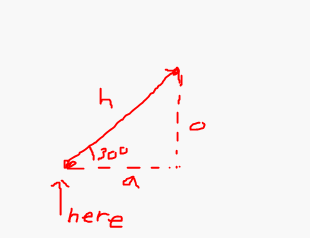# How to apply trigonometry to roblox?

Hello,
I would like to find out how to use trigonometry for roblox. I’ve seen tonnes of cool stuff made from sines and tangents and whatnot, like circular motions, UI physics, ect.
But I am not sure how to use it at all. I have personally never studied trigonometry at home or at school, I know a BIT about the basics like how you can find sines with hypotenuse or whatever but I have never really found out any use for it in roblox or other coding. Help me.
- Br, iSyriux

2 Likes

It can be used, but it’s not required
For example, if you want to move a part 50 studs away, at an angle of 30 degrees

If you know the basics, you probably know these

cos(angle) = a/h
sin(angle) = o/h

You can do a few manipulations and do something like this

a = cos(angle) * h
o = sin(angle) * h

The adjacent side can be the X value, and the opposing side can be the Y value``````local ANGLE = 30
local DISTANCE = 50

local originalPosition = part.Position
local radian = math.rad(ANGLE) -- math.sin and math.cos take a radian and not a degree, so we have to convert it

local newPosition = originalPosition + Vector3.new(math.cos(radian) * DISTANCE, 0, math.sin(radian) * DISTANCE)

part.Position = newPosition
``````

However, you can easily multiply CFrames, so using trigonometry in this case is not required.

3 Likes